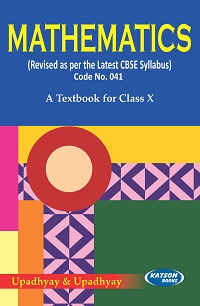•## Mathematics (CBSE) (Class - X)

In Stock
Rs 595.00 Rs 536.00

#### CONTENTS

• Real Numbers
• Polynomials
• Pair of Linear Equations in Two Variables
• Quadratic Equations • Arithmetic Progressions
• Triangles
• Coordinate Geometry
• Introduction to Trigonometry
• Some Applications of Trigonometry
• Circles • Constructions
• Area Related to Circles
• Surface Areas and Volumes
• Statistics
• Probability.

• Publisher : S.K. Kataria & Sons